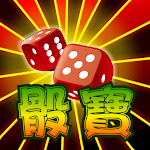ID: com.Sapp.SicBo

• Author:

• Version:

Varies with device

• Update on:

## The description of 骰寶 SicBo 賭大小 Casino

In Sic Bo, each player places a bet on the dealer. Before each bet, the dealer puts three dice in a covered vessel and shakes. When each player has finished betting, the dealer opens the vessel and pays out. Because the most common bet is to buy the size of the dice points (the total number of points is 3 to 10 is called the small, 11 to 18 is the big, except for the dice), it is also often called the buy size (Tai-Sai).

In addition to the categories mentioned below, some casinos can also bet on singles, doubles (calculated by the addition of three points), specific three points of any three dice, etc.

Bet size:
Big: The sum of the points of the three dice is between 11 and 17.
Small: The sum of the points of the three dice is between 4 and 10.

(In case of Wai dice, the dealer takes all)

Betting on Odd and Double:
Single: The sum of points is a single.

Double: The sum of points is double.
(In case of Wai dice, the dealer takes all)

Betting number:
Three dice roll the result, hit the number among them.

Round dice:
The three dice bet have the same number.

Whole circumference:
Bets on any three numbers of the same dice.

Single dice dice surface:
All six numbers can be bet on, according to the three dice, the selected number and the number of times the dice appear is the winning, which is calculated according to the odds.